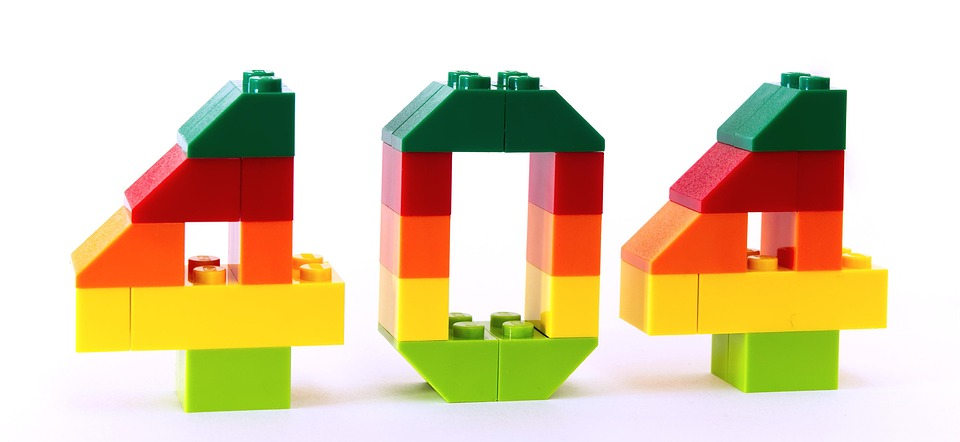# 7.1 Introducing Statistics: Should I Worry About Error?

#type1error

#type2error

#bias

#statisticalinference

written byjosh argoimage courtesy of: pixabay

## What Causes Statistical Error

No statistical study is perfect. There is always a chance that some sort of error occurs. This may be due to bias in sampling, questioning or other confounding variables within the statistical study.

## Type 1 Error

A type 1 error is an error in which we reject the null hypothesis presented by a given claim in favor of an alternative hypothesis erroneously. The probability of a Type 1 error occurring is always equal to the alpha/significance level of the study (usually 0.05).

### Example

Let's say an author claims that the mean income for a given area is \$45,000.

We sample a group of 50 families and find that the mean income of our sample is \$60,000 with a standard deviation of \$2,500. In performing a statistical test, we would reject the author's claim. If we made an error in our study (either due to sampling or random chance), this would be a Type 1 error.

## Type 2 Errors

A type 2 error is an error in which we fail to reject the null hypothesis presented by a given claim erroneously. Instead, we rejected the null hypothesis, when in reality, it should not have been rejected. Type 2 errors are common in statistical studies where we have a small sample size.

### Example

Let's say an author claims that the mean income for a given area is \$45,000.

We sample a group of 50 families and find that the mean income of our sample is \$44,500 with a standard deviation of \$1,000. In performing a statistical test, we would fail to reject the author's claim. If we made an error in our study (either due to sampling or by random chance), this would be a Type 2 error.

## How to Minimize Error in Statistical Studies

### Minimizing error due to bias in sampling

✔️ Select a random sample using a method such as simple random sampling.

• Example: A band director wants to survey the school on their opinions of this year's half-time show. To perform the survey, he numbers each student in the school with a number and uses a random number generator to select 20 students. This is a GOOD example of how to select a random sample.

❌ Avoid volunteer samples, convenience samples and other sampling methods that may heavily influence your data in one direction.

• Example: A band director wants to survey the school on their opinions of this year's half-time show. To perform the survey, he chooses the first 20 students who arrive at the Fall Band Concert and asks them if the band's show is satisfactory. This is a BAD example of how to select a random sample since he is only using students who were coming to the band concert (convenience sample).

### Minimizing error due to bias in questioning

❌Avoid asking questions in a way that will prompt a certain response

• Example: If the band director wants to know how the half-time show was, he should ask the question in a way such as "Rate the band's half time show on a scale of 1-10" as opposed to saying, "Was the band's half-time show good?" The latter way of asking the question will likely influence the student's to say "yes". This is known as response bias.

❌Avoid having someone ask questions that may influence the response (for example, don't have a police officer ask someone if they have ever broken the speed limit)

• Example: If the band director wants to know how the half-time show was, an anonymous survey would be the best way. If the band director asked the students directly, they may feel more inclined to give it a higher rating (especially if their grade in class could be influenced by the way they feel).

### Minimizing error due to confounding variables

✔️Use blocking in your experiment to account for any known or suspected confounding variables.

• Example: If the band director wants to know how the student body feels about the half-time show, the band director may consider blocking by grade. That would ensure an equal amount of responses from each class and make sure that the age of students was not a confounding variable.

🎥Watch: AP Stats - Errors and Powers of Tests